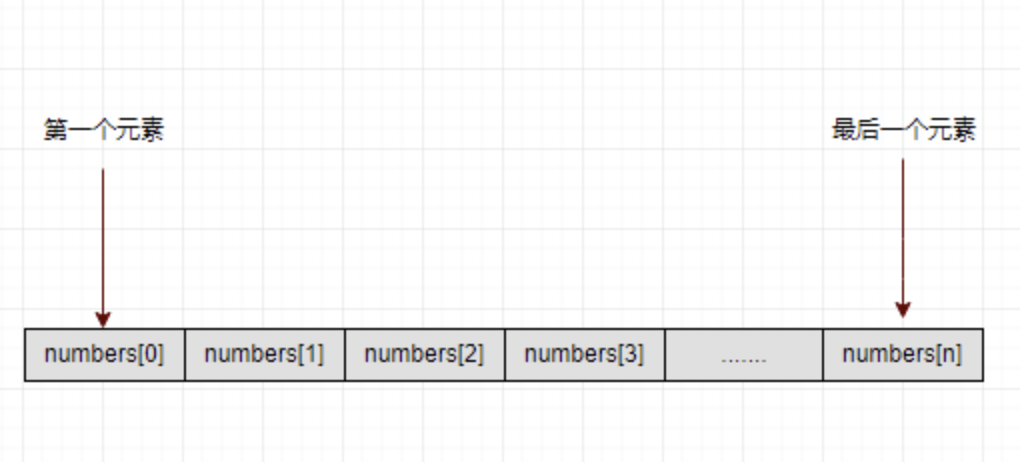详解C语言的C#数组

数组是一种存储相同类型元素的固定大小顺序集合。数组用于存储数据集合，但一般会将数组视为存储在连续存储器位置的相同类型的变量的集合。

如果要存储表示100名称学生的分数，需要独立地声明100整数变量。例如：number0.number1...., number100这样单个独立变量。而如果使用一个数组变量来表示就省事多了。例如，首先声明数组：numbers，使用numbers，numbers和...，numbers来表示单个变量，数组中的元素可通过索引来访问。

所有数组是由连续的内存位置组成。最低的地址对应于第一个元素，而最后一个元素的地址最高。声明数组

要在 C# 中声明一个数组，可以使用以下语法：

datatype[] arrayName;

其中 ：

datatype ： 用于指定数组中元素的类型。

[] ： 指定数组序号，rank指定数组的大小。

arrayName ： 指定数组的名称。

例如，

double[] balance;

初始化数组

声明数组不会将的数组初始化到内存中。将数组变量初始化时，可以为数组指定值。

数组是一个引用类型，因此需要使用new关键字来创建数组的实例。 例如，

double[] balance = new double;

数组赋值

可以通过使用索引数为各个数组元素分配值，如：

double[] balance = new double;
balance = 1500.0;
balance = 1000.0;
balance = 2000.0;

也可以在声明时为数组指定值，如下所示：

double[] balance = { 240.08, 523.19, 121.01};

还可以在创建时初始化数组，如下所示：

int [] marks = new int  { 89,  98, 97, 87, 85};

也可以省略数组的大小，如下所示：

int [] marks = new int[]  { 100,  97, 96, 97, 95};

可以将数组变量复制到另一个目标数组变量中。在这种情况下，目标和源都指向相同的内存位置：

int [] marks = new int[]  { 99,  98, 92, 97, 95};
int[] score = marks;

创建数组时， C# 编译器会根据数组类型将每个数组元素初始化为默认值。 例如，对于int类型的数组，所有元素都将初始化为0.

访问数组元素

通过索引和数组名称来访问数组的元素。这是通过将元素的索引放在数组的名称后面的方括号内完成的。 例如，

double salary = balance;

以下示例演示了如何声明，赋值和访问数组：

using System;
namespace ArrayApplication
{
class MyArray
{
static void Main(string[] args)
{
int []  n = new int; /* n is an array of 10 integers */
int i,j;

/* initialize elements of array n */
for ( i = 0; i < 10; i++ )
{
n[ i ] = i + 100;
}

/* output each array element's value */
for (j = 0; j < 10; j++ )
{
Console.WriteLine("Element[{0}] = {1}", j, n[j]);
}
}
}
}

当编译和执行上述代码时，会产生以下结果：

Element = 100
Element = 101
Element = 102
Element = 103
Element = 104
Element = 105
Element = 106
Element = 107
Element = 108
Element = 109

使用foreach循环

在前面的例子中，我们使用for循环访问每个数组元素。还可以使用foreach语句来遍历数组。参考以下代码 ：

using System;
namespace ArrayApplication
{
class MyArray
{
static void Main(string[] args)
{
int []  n = new int; /* n is an array of 10 integers */

/* initialize elements of array n */
for ( int i = 0; i < 10; i++ )
{
n[i] = i + 100;
}

/* output each array element's value */
foreach (int j in n )
{
int i = j-100;
Console.WriteLine("Element[{0}] = {1}", i, j);

}
}
}
}

当编译和执行上述代码时，会产生以下结果：

Element = 100
Element = 101
Element = 102
Element = 103
Element = 104
Element = 105
Element = 106
Element = 107
Element = 108
Element = 109

C# 数组类型

C# 程序员应该要清楚以下几个与数组有关的重要概念：

Array类

System命名空间中定义，它是所有数组的基类，

以上就是小编为大家整理发布的“详解C语言的C#数组”一文，更多相关内容尽在开课吧广场C++教程频道。有用1分享